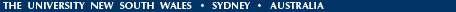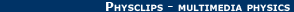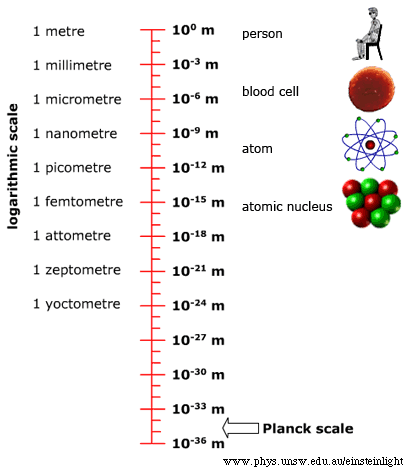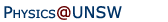Relativity in brief... or in detail..

# The Planck scale: relativity meets quantum mechanics meets gravity.

The Planck scale combines the quantities used in relativity, quantum mechanics and gravity (c, h and G). On the Planck scale, simple ideas about time and space break down. However, the Planck units appear to give absolute scales of length and time. This poses important challenges for Special Relativity, as this page explains, following a brief introduction to the Planck scale.
• Does Planck-scale physics challenge relativity?
• Defining the Planck scale
• Combining quantum mechanics, gravity and relativity
• What do space and time mean at the Planck scale?

Does Planck-scale physics challenge relativity? According to Special Relativity's time dilation and length contraction, two observers with relative motion are condemned to eternal disagreement about times and lengths. Or are they? They will disagree over the arbitrary times measured in seconds and lengths measured in metres or whatever units. They would also disagree over clock ticks, atomic vibrations and light wavelengths. But what if there were some absolute time and length, determined by the very nature of the universe? And what if these quantities could be measured in different frames of reference? Wouldn't the observers have to agree on those having the same value, if they agree on the same laws of physics?

Well, there is an abolute length and an absolute time. It's possible that we'll never be able to measure them, so any threat posed to relativity is distant and perhaps even hypothetical. It is interesting to see whether and how these quantities might be included in relativity.### Defining the Planck scale

The Planck length LP is defined by taking the constants of nature and combining them in such a way that their units combine to give a length. Planck's constant, h, has units of joule seconds. (These days, physicists usually work with h/2π, and so shall we.) Newton's constant G (the constant of gravitation) has units of N.m2kg-2. And the speed of light c has units of m.s-1. Check for yourself that the combination that gives length is:
LP = (hG/2πc3)1/2 .
This defines the Planck length, which is 1.6 x 10-35 metres. (That's 0.000 000 000 000 000 000 000 000 000 000 000 016 meters.) To give you an idea, let's compare it with the size of an atom, which is already about 100,000 times smaller than anything you can see with your unaided eye (an atom size is about 0.000 000 0001 meters). Suppose that you measured the diameter of an atom in Planck lengths, and that you counted off one Planck length every second. To measure the atomic diameter in Planck lengths would take you 10,000,000 times the current age of the universe. (After which time, for Joyce fans, the fires of hell may well have begun to begin to burn.)

Divide the minuscule Planck length by the speed of light (which is pretty big) and you get a really tiny unit of time, the Planck time, tP, which is:
tP = (hG/2πc5)1/2 .
The Planck time is 5.4 x 10-44 seconds. (I'm wearing out the zero key on this keyboard, so I shan't write it out in full but you get the idea: it's brief.)

There is also a Planck mass, which is (ch/2πG)1/2 = 22 μg. This doesn't sound very much, until you think of a fundamental particle with that mass. Or until you convert it into energy by multiplying by c2 to get 2.0 109 joules or 1.2 x 1028 eV. Yes, 2 billion joules all concentrated in one fundamental particle.

So, the Planck length and time are very small, but they are results solely of the values that appear naturally in our physical laws. So according to the principle of Special Relativity, it seems that observers in different frames should observe the Planck length and time to be the same. So what about time dilation and length contraction? If—and it's a very big if when you think about it—if these lengths and times are observable as physical lengths and intervals in moving frames, it appears that we shall need to modify Special Relativity to include them. One theory that does so is called Doubly Special Relativity, suggested in 2002 by Giovanni Amelino-Camelia.

Before we get too excited, we should point out just how far beyond current experimental technology these effects are. Particle accelerators are described by the energies that they can produce, and the latest generation produces energies of ~10 TeV, or 1013 eV. The Planck energy is 1.2 x 1028 eV. We are short by a factor of 1015.

Further, it's not clear (to this author, at least) what it would mean to measure these lengths and times in or from different frames of reference. As we'll see below, on the Planck scale, time and space no longer have their ordinary, macroscopic meaning and so naïve applications of relativity are probably inappropriate.

### Combining quantum mechanics, gravity and relativity

So, where do the Planck scale quantities come from? The speed of light c is the natural unit that relates time and space. G is the constant of gravity, and h is Planck's constant, which comes from quantum mechanics. So the Planck scale defines the meeting point of gravity, quantum mechanics, time and space. Currently, we don't know much about this interaction, because gravity is so feeble that its influence on things as small as quantum systems is immeasrably small — or alternatively we could say that quantum effects are immeasurably small for objects large enough to have substantial gravitational effects.

Special Relativity and quantum mechanics work very well together. Indeed, relativistic quantum electrodynamic is a spectacularly accurate theory. Richard Feynman once described how accurate it was by saying: if as though you asked me how far it was to the moon and I said "do you mean from my head or from my feet?" And it's now more accurate than when he said that.### What do space and time mean at the Planck scale?

Quantum mechanics and gravity (whether Newton's theory of gravity or Einstein's theory of General Relativity) do not fit so neatly together. The problem can be put in several different ways, but I favour this one. From our discussion of virtual particles (Why there would be no chemistry without relativity), we saw that virtual particles could be more massive if their lifetime and range were smaller.

Now both Newton's and Einstein's gravity predict that enough mass in a small enough space can produce a black hole: a region with a gravitational field so strong that its escape velocity is greater than c. When we put the two ideas together, we find that there is a scale small enough for virtual black holes to exist. This is the Planck scale. On this scale, a black hole can appear spontaneously — but very briefly — anywhere in space. So, examined on this scale, all of the weird, singular behaviour associated with black holes asserts itself, not just in remote astrophysical peculiarities, but everywhere and at all times. With black holes winking in and out of existence everywhere, space and time as continuous entities cease to have meanings when discussing distances of 10-35 metres and times of 10-44 seconds. So relativity, a classical theory of space and time based on a space-time continuum, might run into serious difficulties.

Which is perhaps not surprising: the Planck scale is a very, very long extrapolation from our current knowledge. And from our current cosmological era. When the age of the universe was ~tP, its temperature was ~EP, and Planck scale phenomena dominated.

On this topic, we have so little direct knowledge that there are few hints to guide the development of theories, and few constraints upon those theories. Currently, there are several different families of theories that aim to produce a consistent theory of quantum gravity. Some include a larger number of spatial dimensions, not all of which are macroscopic*. At the moment, however interesting they be, these theories are speculative. Perhaps one of them will turn out to be a good, useful theory, and the others will fall. At the moment, we cannot put them to the test.

Today, we remember Democritus for speculating on the existence of atoms. But the ancient Greek philosophers proposed so many ideas that it is perhaps not too surprising that some of them turned out to be consistent with facts determined much later. Meanwhile, here are a couple of our pages that may interest you:

* How can we have more than three spatial dimensions? Surely Gauss' law (for electricity, magnetism and gravity) shows that we live in a locally flat geometry with three spatial dimensions?

Yes, experiments to test Gauss' law either directly or indirectly do show that our geometry is both very flat and three dimensional on the scale of the experiments conducted. If, however, the universe were closed in all but three of the spatial dimensions, and if, in the closed dimensions, the radius of the universe were much smaller than the size of measurements (say less than the dimsions of fundamental particles), then Gauss' law would apply only to the three large dimensions — a Gaussian surface would never intersect one of the closed dimensions. Consequently electricity, magnetism and gravity would be inverse square laws on the scales that are experimentally accessible. The theories that use extra dimensions then have the possibility to use such ideas as representing particles as standing waves on the circumference of the universe in the closed dimensions.

•Home | Summary | Quiz | Credits School of Physics - The University of New South Wales Sydney, Australia 2052 © School of Physics UNSW 2052 Disclaimer feedback